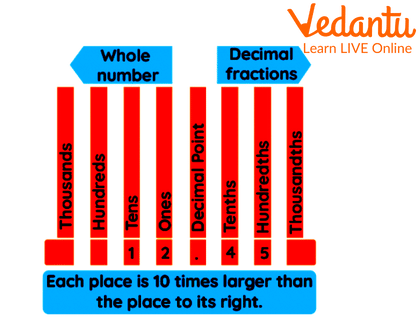Courses
Courses for Kids
Free study material
Free LIVE classes
More

# Word Problems On DecimalsLIVE
Join Vedantu’s FREE Mastercalss

## Introduction

Decimals are numbers that fall between integers on a number line. In mathematics, they are just another method to express fractions. We may write more accurate values of quantifiable things such as length, weight, distance, money, and so on using decimals.

## Define Decimals

Decimals is the exact value of the fraction which contains both whole numbers as well as fraction parts in them. The decimal number can be identified by the “.” Or a decimal sign in between the digits in the number. The numbers towards the left of the decimal sign are integers or whole numbers, while the numbers to the right are decimal fractions. . If we proceed directly from one place, the following place will be ( $\frac{1}{{10}}$ ) times smaller, resulting in a ( $\frac{1}{{10}}$ )th or tenth place value. Take a look at the decimal place value chart for the number 12.45, for example.Place Value Chart Of Decimal Number

## Addition And Subtraction Of Decimals

The standard addition and subtraction rules apply when adding and subtracting decimals. The only details to consider are the decimal places following the decimal point. The numbers must be put in columns based on where they fall before and after the decimal point. According to the decimal place value chart, place values before the decimal point begin with ones, tens, hundreds, and so on, but place values following the decimal point begin with tenths, followed by hundredths, and so on.

## Example Of Addition Word Problems Of Decimals

Sample problem: you brought two erasers of 2.25 and 3.89 bucks each. How much money did you spend on the erasers?

Ans:

Step one: write the decimal number under their columns of their own place values.

Step two: add the digits present in the same column.

Money spent on first eraser = 2.25

Money spent on second eraser= 3.89

Total money spent on the erasers = 6.14

 O. t h 1 (carry over) 1 (carry over) 2. 2 5 $+$ 3. 8 9 6. 1 4

## Example Of Subtraction Of Decimal Number

Sample problem: Ritu had 500 gm of flour with her. She used 250.78gm of flour to bake a cake. How much flour is left with her?

Ans:

Step one: write the digits in their place value columns.

Step two: Subtract the digits placed in the same column.

Total amount of flour = 500gm

Amount of flour used for baking cake = 250.78gm

Amount of flour left= 249.22gm

H

T

O.

t

h

4

9

9

9

10(borrow)

5

0

0.

0

0

$-$ 2

5

0.

7

8

2

4

9.

2

2

## Conclusion

Decimals can be added, subtracted, multiplied and divided. Putting a zero after the decimal digits has no effect on the value of a decimal. For instance, 5 can be written as 5.00. Despite the fact that the time and angle measurements are in decimal format, those cannot be subtracted or added together as decimals.

## Decimal Word Problems With Solutions

1. Kavita travelled 267.97km and Sheena travelled 862.73km using their own cars. Who travelled the most and by how much?

a. 590.74, Kavita

b. 594.76, Sheena

c. 549.67, Sheena

d. 509.47, Kavita

Ans: 594.76, Sheena

Explanation: the approximate distance covered by Kavita is 200km and Sheena is 800km. As the distance travelled by Sheena is the longest so we will subtract 862.73 from 267.97 which will give us the answer as 594.76.

 H T O. t h BORROW 15 11 16 13 7 6 2. 7 3 $-$ 2 6 7. 9 7 5 9 4. 7 6

2. Annie spent 29.89 bucks on fruits and 62.45 bucks on vegetables. How much money did she spend in total?

a. 92.34

b. 92.35

c. 93.72

d. 90.45

Ans: 92.34

Explanation: the amount of money spent on vegetables and fruits can be calculated by adding the amount spent on each item. So, the digits would be placed in their columns and would be added together to get the result.

 T O. t h 1 1 1 carryover 2 9. 8 9 $+$ 6 2. 4 5 9 2. 3 4

3. The cost of mangoes is 85.345 and the cost of apples is two times that of mangoes then what is the cost of apples?

a. 170.69

b. 170.89

c. 170.79

d. 170.99

Ans: 170.69

Explanation: in this question, we need to do multiplication to find the original cost of the apples. For that, you need to multiply 85.345 by 2.

 T O. t h th 1 1 carryover 8 5. 3 4 5 $\times$ 2. 0 0 0 1 7 0. 6 9 0

Last updated date: 01st Oct 2023
Total views: 82.2k
Views today: 1.81k

## FAQs on Word Problems On Decimals

1. What are non-decimal numbers?

The numbers that do not have a fractional part in them are called non-decimal numbers.

2. Can a decimal number be considered an integer?

The integer numbers can be changed into decimal form by placing 0 after the decimal point. But the decimal numbers cannot be changed into integers. So decimals are not integers.

3. What are the uses of decimals?

Decimals are used in everyday life as they are used to measure the exact amount of money, weight and length of objects.

4. What are repeating decimals?

The numbers having the same digit which are repeated after the decimal point are the repeating decimal numbers.

5. How Do You Divide One Decimal By Another?

To divide a decimal number by a decimal number, we must first convert the divisor to a whole number and then proceed with the division.

Step one: Change the decimal number into a whole number by multiplying it by 10,100, or 1000 depending on the digits present after the decimal point.

Step two: Now divide the whole numbers like the standard division.